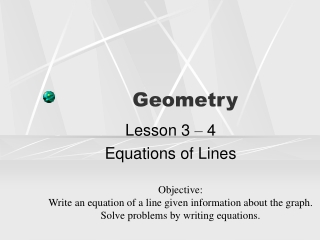DownloadDownload PresentationGeometry

# Geometry

Download Presentation## Geometry

- - - - - - - - - - - - - - - - - - - - - - - - - - - E N D - - - - - - - - - - - - - - - - - - - - - - - - - - -
##### Presentation Transcript

1. Geometry Lesson 3 – 4 Equations of Lines Objective: Write an equation of a line given information about the graph. Solve problems by writing equations.

2. Equation Forms • Slope-Intercept Form • y = mx + b • m – slope • b – y-intercept • Point-Slope Form • y – y1 = m(x – x1) • m – slope • (x1, y1)

3. Write an equation in slope-intercept form of the line with slope 3 and y-intercept of –2. Then graph the line. • y = mx + b • y = 3x – 2 • Graphing: • Put a dot on the y-intercept • (0, 3) • Use slope (rise/run) to find more points. • Up 3 over 1

4. Write an equation in slope-intercept form of the line with slope ½ and y-intercept of 8. Then graph the line. • y = ½ x + 8 • Graphing • Graph (0, 8) • Up 1 over 2 Don’t have to go by 2’s

5. Write an equation in point-slope form of the line with slope –3/4 that contains (-2,5). Then graph. • y – y1 = m(x – x1) (x – (-2)) Graphing: plot point, then use slope

6. Write an equation in point-slope of the line with slope of 4 that contains (-3, -6). Then graph. • y + 6 = 4(x + 3)

7. Method 1: Find the slope m = 2 Use slope-intercept form Pick one of the points 3 = 2(0) + b b = 3 y = 2x + 3 Method 2: Find the slope m = 2 Use point-slope form Pick one of the points y – 3 = 2(x – 0) Solve for y to put in slope-intercept form. y – 3 = 2(x – 0) y – 3 = 2x y = 2x + 3 Write an equation of the line through each points in slope-intercept form(0, 3) and (-2, -1) You may use either method

8. Write an equation of the line through each points in slope-intercept form(-2, 4) & (8, 10)

9. Write an equation of the line through (-2, 6) & (5, 6) in slope-intercept form. • Find the slope • m = 0 (horizontal line) • 6 = 0(-2) + b • 6 = b • y = 0x + 6 • y = 6 Make sure you simplify. Do not leave 0x or 0

10. Horizontal & Vertical Lines • When an equation only has 1 variable it is either a horizontal or vertical line • The equation tells you how to graph. • y = 6 • The line goes through the axis of the variable given. • A horizontal line at 6 through the y-axis.

11. y = 3 x = 6 x = -1 5 = y -9 = x x = 0 y = 0 Tell whether the following is a horizontal or vertical line. horizontal horizontal vertical vertical Vertical (y-axis) vertical Horizontal X-axis

12. Write an equation in slope-intercept form for a line perpendicular to the line y = -3x + 2 through (4, 0). • Step 1: Identify slope for new equation • m = 1/3 (because of perpendicular) • Write new equation with new slope and point.

13. Write an equation is slope-intercept form for a line parallel to y = (-3/4)x + 3 containing (-3, 6) • Step 1: Identify slope for new equation • (-3/4) same slope since parallel • Write new equation with new slope and point.

14. Homework • Pg. 200 1 – 12 all, 14 – 40 E